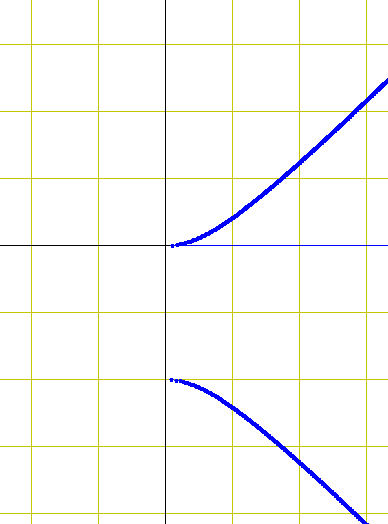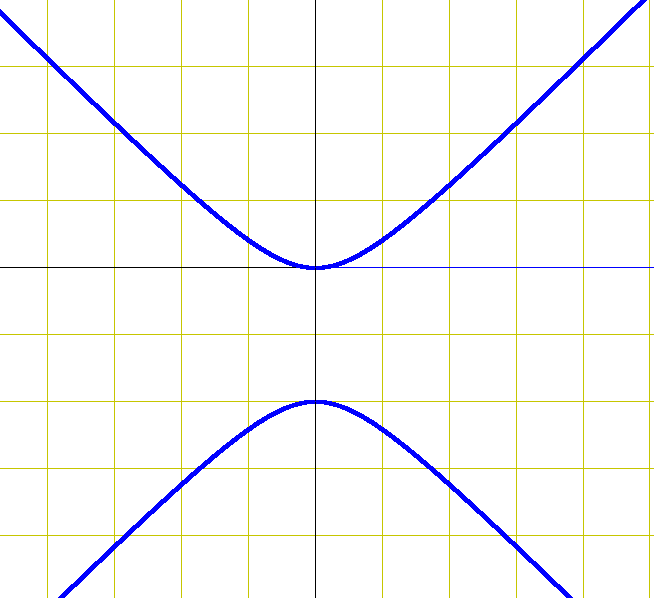# Find the volume of th eresulting solid by and method

• tony873004
In summary, the conversation discusses finding the volume of a solid formed by rotating a region bounded by a curve about a specified axis. The curve is given as x^2 + (y-1)^2 = 1 and the question asks for the volume when rotated about the x-axis and the y-axis. The conversation also discusses the difficulty in graphing the curve and solving for x in terms of y. Eventually, the correct solution is found, resulting in a unit sphere with a volume of 4pi/3.

#### tony873004

Gold Member
The region bounded by the curves is rotated about the specified axis. Find the volume of th eresulting solid by and method.

$$x^2 + \left( {y - 1} \right)^2 = 1$$

First question want it rotated about the x-axis, the second question wants it rotated about the y-axis.

So I re-write it in terms of x. Seems easier than in terms of y since there's only 1 x term.
$$x = \sqrt { - y^2 + 2y}$$

The problem is when I graph it,So nothing is bounded. There is no solid to rotate. Should I have solved in terms of y instead? How would I do that?

Last edited:
Don't you need to take both the positive and negative square roots?

cepheid said:
Don't you need to take both the positive and negative square roots?

It still seems like there is no bounded region. All the other problems in this section give a second curve, usually a line like y=2, just to bound the curve. What am I doing wrong? Did I properly solve for x?You have lost a "sign". x2+ (y-1)2= 1 is obviously a circle of radius 1 with center at (0, 1), not the hyperbola you show.

$$x= \pm \sqrt{1-(y-1)^2}= \pm \sqrt{2y- y^2}$$
and
$$y= 1\pm \sqrt{1- x^2}$$

lol... and I should have recognized that the answer in the back of the book, 4pi/3 is the volume of a unit sphere. Without the r^3 in the formula I didn't recognize it.

Thanks, Halls